# 来吧！动手搭建一个基于 CNN 的电影推荐系统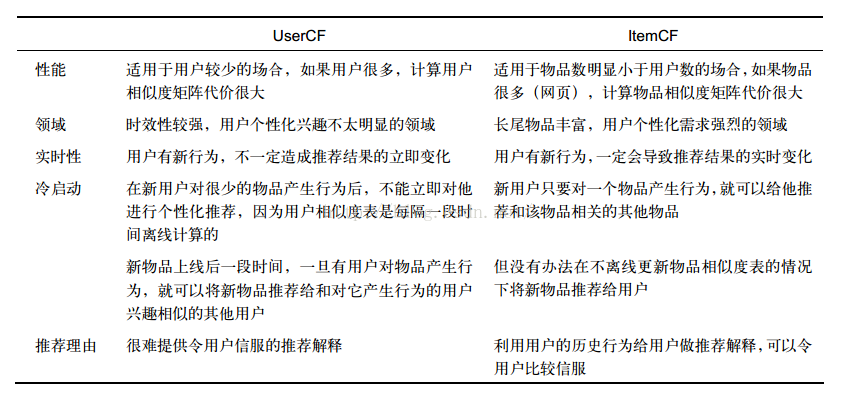CNN 是如何应用在文本处理上的？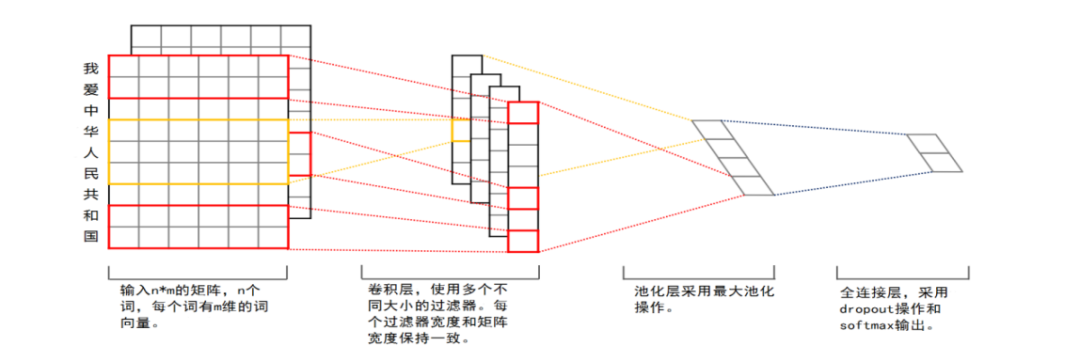https://github.com/chengstone/movie_recommender

1. 定义用户嵌入矩阵
用户的特征矩阵主要是通过用户信息嵌入网络来生成的，在预处理数据的时候，我们将 UserID、MovieID、性别、年龄、职业特征全部转成了数字类型，然后把这个数字当作嵌入矩阵的索引，在网络的第一层就使用嵌入层，这样数据输入的维度保持在（N，32）和（N，16）。然后进行全连接层，转成（N，128）的大小，再进行全连接层，转成（N，200）的大小，这样最后输出的用户特征维度相对比较高，也保证了能把每个用户所带有的特征充分携带并通过特征表达。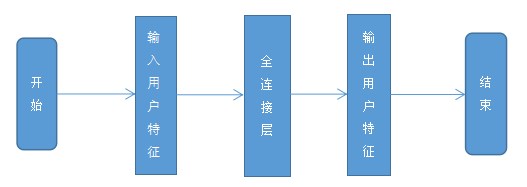1. 生成用户特征
生成用户特征是在用户嵌入矩阵网络输出结果的基础上，通过2层全连接层实现的。第一个全连接层把特征矩阵转成（N，128）的大小，再进行第二次全连接层，转成（N，200）的大小，这样最后输出的用户特征维度相对比较高，也保证了能把每个用户所带有的特征充分携带并通过特征表达。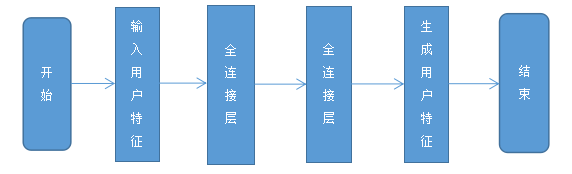1. 定义电影 ID 嵌入矩阵
通过电影 ID 和电影类型分别生成电影 ID 和电影类型特征，电影类型的多个嵌入向量做加和输出。电影 ID 的实现过程和上面一样，但是对于电影类型的处理相较于上面，稍微复杂一点。因为电影类型有重叠性，一个电影可以属于多个类别，当把电影类型从嵌入矩阵索引出来之后是一个（N，32）形状的矩阵，因为有多个类别，这里采用的处理方式是矩阵求和，把类别加上去，变成（1，32）形状，这样使得电影的类别信息不会丢失。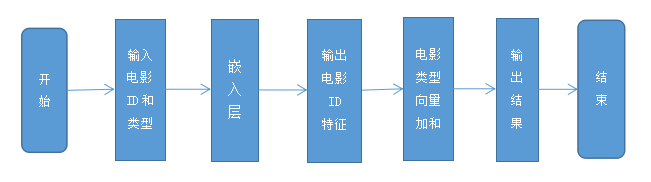1. 文本卷积神经网络设计
文本卷积神经网络和单纯的 CNN 网络结构有点不同，因为自然语言通常是一段文字与图片像素组成的矩阵是不一样的。在电影文本特征矩阵中，矩阵的每一个行构成的行向量代表一个 Token，包括词或者字符。如果一段文字有 n 个词，每个词有 m 维的词向量，那么我们可以构造出一个 n*m 的矩阵。而且 NLP 处理过程中，会有多个不同大小的过滤器串行执行，且过滤器宽度和矩阵宽度保持一致，是整行滑动。在执行完卷积操作之后采用了 ReLU 激活函数，然后采用最大池化操作，最后通过全连接并 Dropout 操作和 Softmax 输出。这里电影名称的处理比较特殊，并没有采用循环神经网络，而采用的是文本在 CNN 网络上的应用。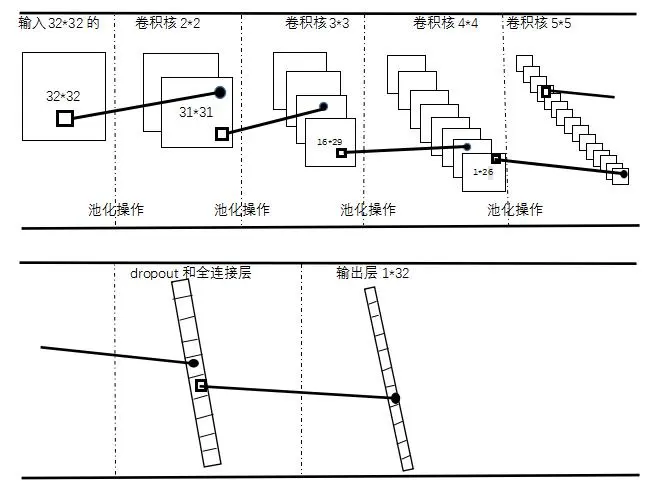（1）首先输入一个 32*32 的矩阵；

（2）第一次卷积核大小为 22，得到 3131 的矩阵，然后通过 [1,14,1,1] 的 max-pooling 操作，得到的矩阵为 18*31；

（3）第二次卷积核大小为 33，得到 1629的矩阵，然后通过[1,13,1,1] 的 max-pooling 操作，得到的矩阵为 4*29；

（4）第三次卷积核大小 44，得到 126 的矩阵，然后通过 [1,12,1,1] 的 max-pooling 操作，得到的矩阵为 1*26；

（5）第四次卷积核大小 55，得到 122 的矩阵，然后通过 [1,11,1,1] 的 max-pooling 操作，得到的矩阵为 1*22；

（6）最后通过 Dropout 和全连接层，len(window_sizes) filter_num =32，得到 132的矩阵。

1. 电影各层做一个全连接层
将上面几步生成的特征向量，通过2个全连接层连接在一起，第一个全连接层是电影 ID 特征和电影类型特征先全连接，之后再和 CNN 生成的电影名称特征全连接，生成最后的特征集。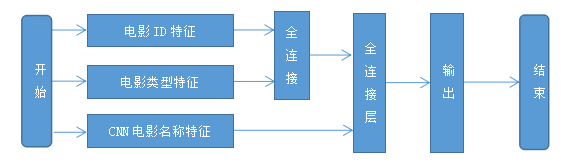1. 完整的基于 CNN 的电影推荐流程
把以上实现的模块组合成整个算法，将网络模型作为回归问题进行训练，得到训练好的用户特征矩阵和电影特征矩阵进行推荐。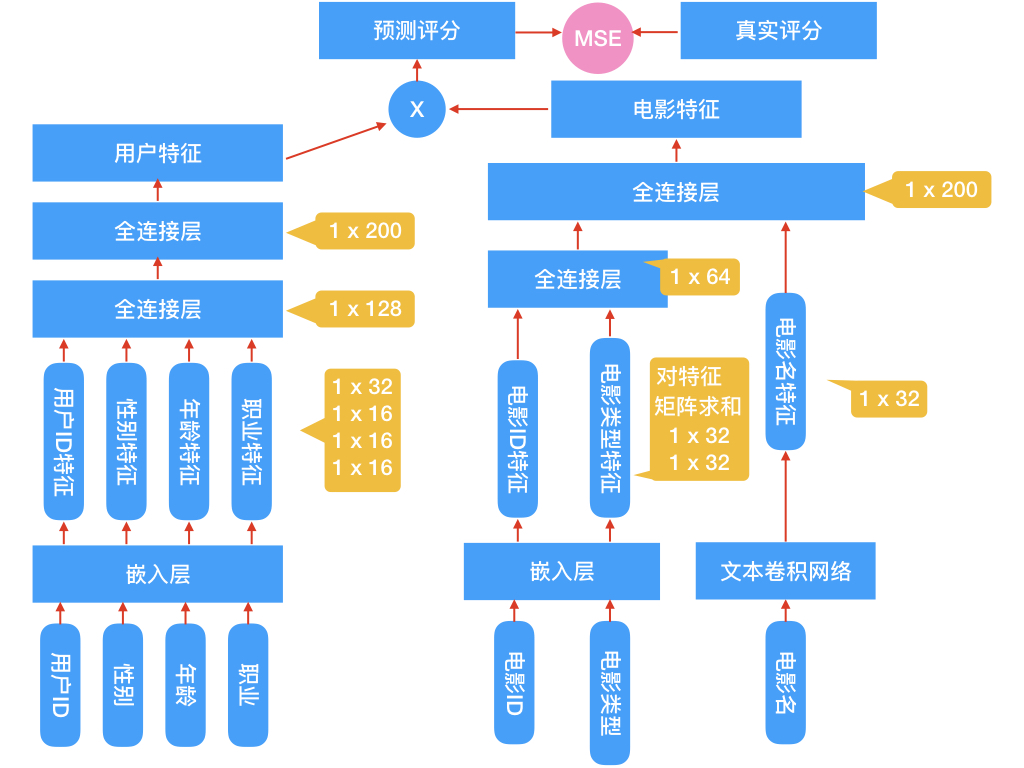# 设置迭代次数
num_epochs = 5
# 设置BatchSize大小
batch_size = 256
#设置dropout保留比例
dropout_keep = 0.5
# 设置学习率
learning_rate = 0.0001
# 设置每轮显示的batches大小
show_every_n_batches = 20


#将数据集分成训练集和测试集，随机种子不固定
train_X,test_X, train_y, test_y = train_test_split(features,
targets_values,
test_size = 0.3,
random_state = 0)

（1）第一步，先固定，learning_rate=0.01 和 num_epochs=10，测试 batch_size=128 对迭代时间和 Loss 的影响；

（2）第二步，先固定，learning_rate=0.01 和 num_epochs=10，测试 batch_size=256 对迭代时间和 Loss 的影响；

（3）第三步，先固定，learning_rate=0.01 和 num_epochs=10，测试 batch_size=512 对迭代时间和 Loss 的影响；

（4）第四步，先固定，learning_rate=0.01 和 num_epochs=5，测试 batch_size=128 对迭代时间和 Loss 的影响；

（5）第五步，先固定，learning_rate=0.01 和 num_epochs=5，测试 batch_size=256 对迭代时间和 Loss 的影响；

（6）第六步，先固定，learning_rate=0.01 和 num_epochs=5，测试 batch_size=512 对迭代时间和 Loss 的影响；

（7）第七步，先固定，batch_size=256 和 num_epochs=5，测试 learning_rate=0.001 对 Loss 的影响；

（8）第八步，先固定，batch_size=256 和 num_epochs=5，测试 learning_rate=0.0005 对 Loss 的影响；

（9）第九步，先固定，batch_size=256 和 num_epochs=5，测试 learning_rate=0.0001 对 Loss 的影响；

（10）第十步，先固定，batch_size=256 和 num_epochs=5，测试 learning_rate=0.00005 对 Loss 的影响。

1. 推荐同类型的电影
思路是：计算当前看的电影特征向量与整个电影特征矩阵的余弦相似度，取相似度最大的 top_k 个，这里加了些随机选择在里面，保证每次的推荐稍稍有些不同。
def recommend_same_type_movie(movie_id_val, top_k = 20):

norm_movie_matrics = tf.sqrt(tf.reduce_sum(tf.square(movie_matrics), 1, keep_dims=True))
normalized_movie_matrics = movie_matrics / norm_movie_matrics

#推荐同类型的电影
probs_embeddings = (movie_matrics[movieid2idx[movie_id_val]]).reshape([1, 200])
probs_similarity = tf.matmul(probs_embeddings, tf.transpose(normalized_movie_matrics))
sim = (probs_similarity.eval())
print("您看的电影是：{}".format(movies_orig[movieid2idx[movie_id_val]]))
print("以下是给您的推荐：")
p = np.squeeze(sim)
p[np.argsort(p)[:-top_k]] = 0
p = p / np.sum(p)
results = set()
while len(results) != 5:
c = np.random.choice(3883, 1, p=p)
for val in (results):
print(val)
print(movies_orig[val])
return result
1. 推荐您喜欢的电影
思路是：使用用户特征向量与电影特征矩阵计算所有电影的评分，取评分最高的 top_k 个，同样加了些随机选择部分。
def recommend_your_favorite_movie(user_id_val, top_k = 10):

#推荐您喜欢的电影
probs_embeddings = (users_matrics[user_id_val-1]).reshape([1, 200])
probs_similarity = tf.matmul(probs_embeddings, tf.transpose(movie_matrics))
sim = (probs_similarity.eval())

print("以下是给您的推荐：")
p = np.squeeze(sim)
p[np.argsort(p)[:-top_k]] = 0
p = p / np.sum(p)
results = set()
while len(results) != 5:
c = np.random.choice(3883, 1, p=p)
for val in (results):
print(val)
print(movies_orig[val])

return results
1. 看过这个电影的人还看了（喜欢）哪些电影
（1）首先选出喜欢某个电影的 top_k 个人，得到这几个人的用户特征向量；

（2）然后计算这几个人对所有电影的评分 ；

（3）选择每个人评分最高的电影作为推荐；

（4）同样加入了随机选择。

def recommend_other_favorite_movie(movie_id_val, top_k = 20):
probs_movie_embeddings = (movie_matrics[movieid2idx[movie_id_val]]).reshape([1, 200])
probs_user_favorite_similarity = tf.matmul(probs_movie_embeddings, tf.transpose(users_matrics))
favorite_user_id = np.argsort(probs_user_favorite_similarity.eval())[-top_k:]

print("您看的电影是：{}".format(movies_orig[movieid2idx[movie_id_val]]))

print("喜欢看这个电影的人是：{}".format(users_orig[favorite_user_id-1]))
probs_users_embeddings = (users_matrics[favorite_user_id-1]).reshape([-1, 200])
probs_similarity = tf.matmul(probs_users_embeddings, tf.transpose(movie_matrics))
sim = (probs_similarity.eval())
p = np.argmax(sim, 1)
print("喜欢看这个电影的人还喜欢看：")
results = set()
while len(results) != 5:
c = p[random.randrange(top_k)]
for val in (results):
print(val)
print(movies_orig[val])
return results

Deep Convolutional Neural Networks for Sentiment Analysis of ShortTexts,CND Santos ,M Gattit ,2014.

【云栖号在线课堂】每天都有产品技术专家分享！

【云栖号在线课堂 社群】https://c.tb.cn/F3.Z8gvnK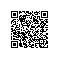使用钉钉扫一扫加入圈子
+ 订阅